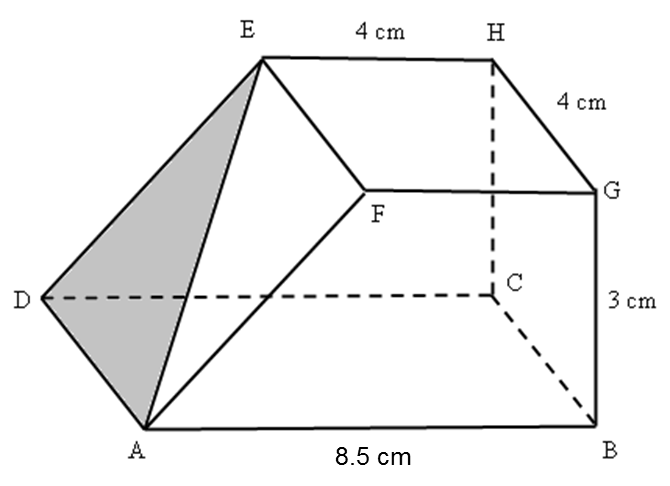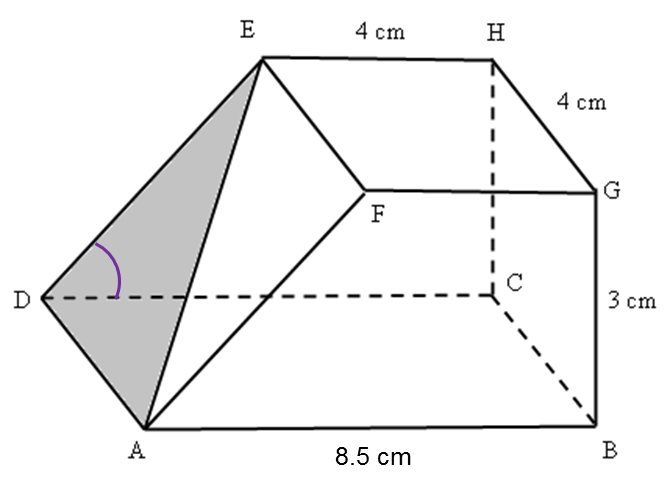# SPM Mathematics (Model Test Paper)

SPM Mathematics (Model Test Paper)

Section A
(52 marks)
Answer all questions in this section.

4 (a) For each of the following statements, determine whether the statement is true or  false.
(i) 35 is multiple of  3 and 5
(ii)   7 is a factor of 42 or 16 is a multiple of 6.
(b)   Write down two implications based on the following sentence:
p3 = –8 if and only if p = –2
(c) Make a general conclusion by induction for a list of numbers 2, 11, 26, 47, …….   which  follows the following pattern.
2 = 3(1)2– 1
11 = 3(2)2– 1
26 = 3(3)2– 1
47 = 3(4)2– 1
…………… [6 marks]

(a)(i) False
(a)(ii) True

(b)
If p3 = –8, then p = –2
If p = –2, then p3 = –8

(c)
3n2– 1, where n = 1, 2, 3, 4, .....

5.   Diagram 2 in the answer space shows a right prism. Trapezium ABGF  is the uniform cross section of the prism.
(a) On Diagram 2, mark the angle between the plane ADE and the plane ADCB.
(b) Calculate the angle between the plane ADE and the plane ADCB.
[3 marks]
(a)Diagram 2

(a)(b)
$\begin{array}{l}\mathrm{tan}\angle EDC=\frac{3}{8.5-4}\\ \text{}=\frac{3}{4.5}\\ \text{}\angle EDC={33.7}^{o}\end{array}$

6.   Diagram 3 shows a right cylinder with a diameter of ( y  + 4 ) cm.Given the volume of the cylinder is 269.5 cm3 and by using  find the value of it’s radius. [4 marks]